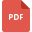# number theory tutorialsSets and their subsets
Mathematics, Grade 7, United States, Number TheoryDistributive Property worksheet
Mathematics, Grade 7, United States, Number TheoryLaw of Indices
Mathematics, Grade 7, United States, Number TheoryCombining Like Terms
Mathematics, Grade 7, United States, Number TheoryScientific Notation
Mathematics, Grade 7, United States, Number TheoryWorksheet on Significant Figures
Mathematics, Grade 8, United States, Number TheoryWriting in Scientific Notation
Mathematics, Grade 7, United States, Number TheoryNegative Indices
Mathematics, Grade 7, United States, Number TheoryCombining Like Terms with properties
Mathematics, Grade 7, United States, Number Theory